# 深入详解 Jetpack Compose | 实现原理

## 深入详解 Jetpack Compose | 实现原理## @Composable 注解意味着什么？

``````// 函数声明
suspend fun MyFun() { … }

// lambda 声明
val myLambda = suspend { … }

// 函数类型
fun MyFun(myParam: suspend () -> Unit) { … }
``````

Kotlin 的 suspend 关键字 适用于处理函数类型：您可以将函数、lambda 或者函数类型声明为 suspend。Compose 与其工作方式相同：它可以改变函数类型。

``````// 函数声明
@Composable fun MyFun() { … }

// lambda 声明
val myLambda = @Composable { … }

// 函数类型
fun MyFun(myParam: @Composable () -> Unit) { … }
``````

``````fun Example(a: () -> Unit, b: suspend () -> Unit) {
a() // 允许
b() // 不允许
}

suspend
fun Example(a: () -> Unit, b: suspend () -> Unit) {
a() // 允许
b() // 允许
}
``````

Composable 的工作方式与其相同。这是因为我们需要一个贯穿所有的上下文调用对象。

``````fun Example(a: () -> Unit, b: @Composable () -> Unit) {
a() // 允许
b() // 不允许
}

@Composable

fun Example(a: () -> Unit, b: @Composable () -> Unit) {
a() // 允许
b() // 允许
}
``````

## 执行模式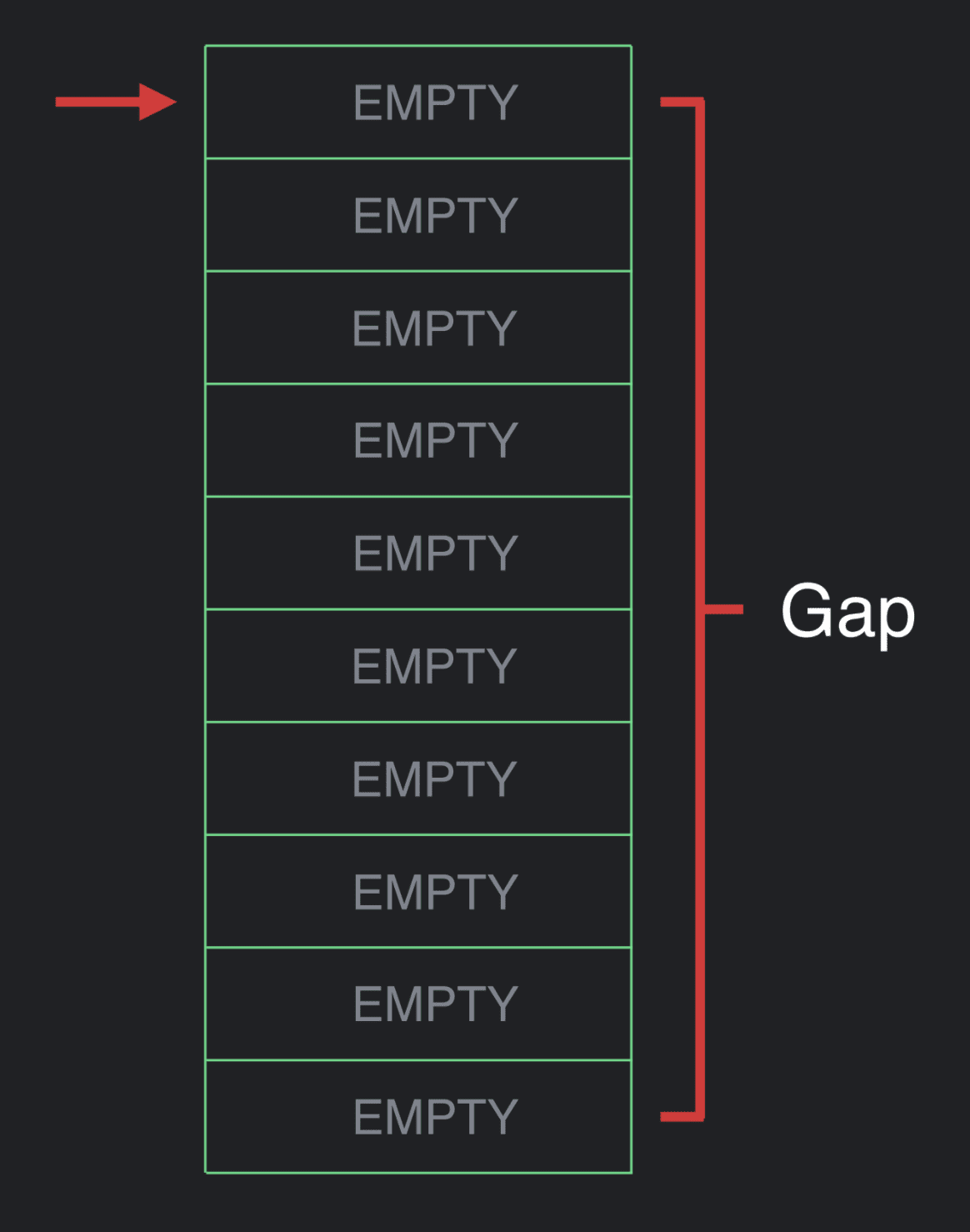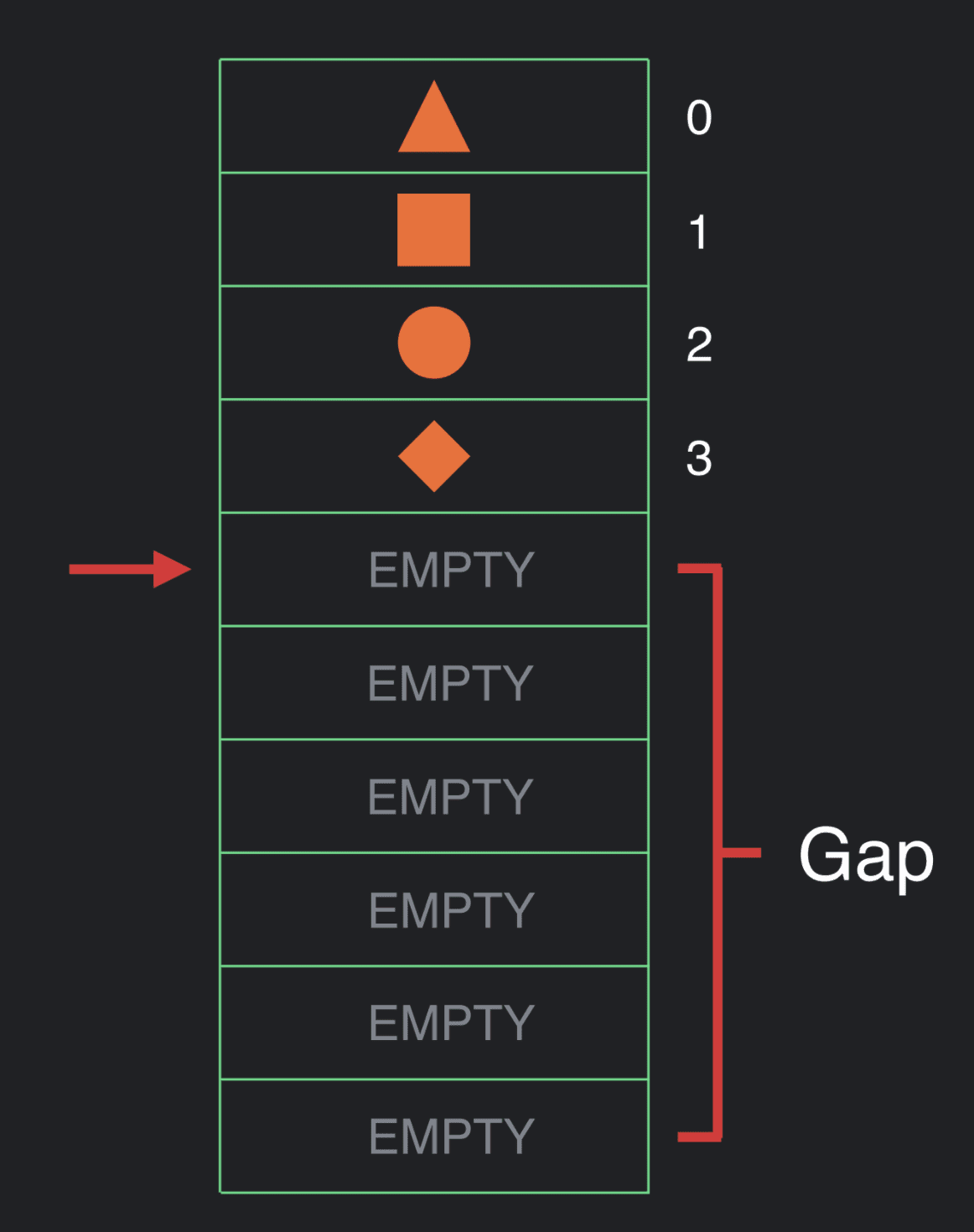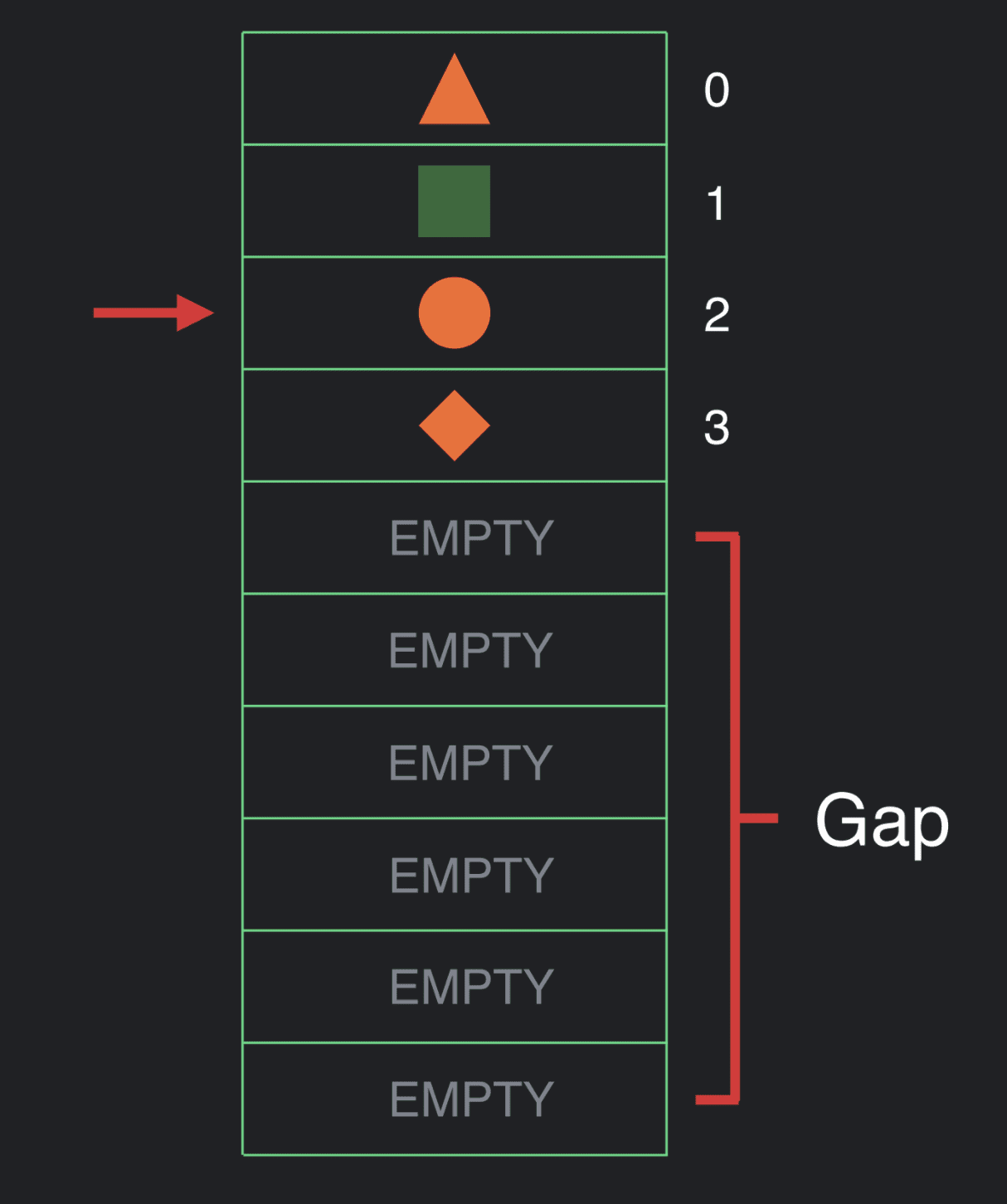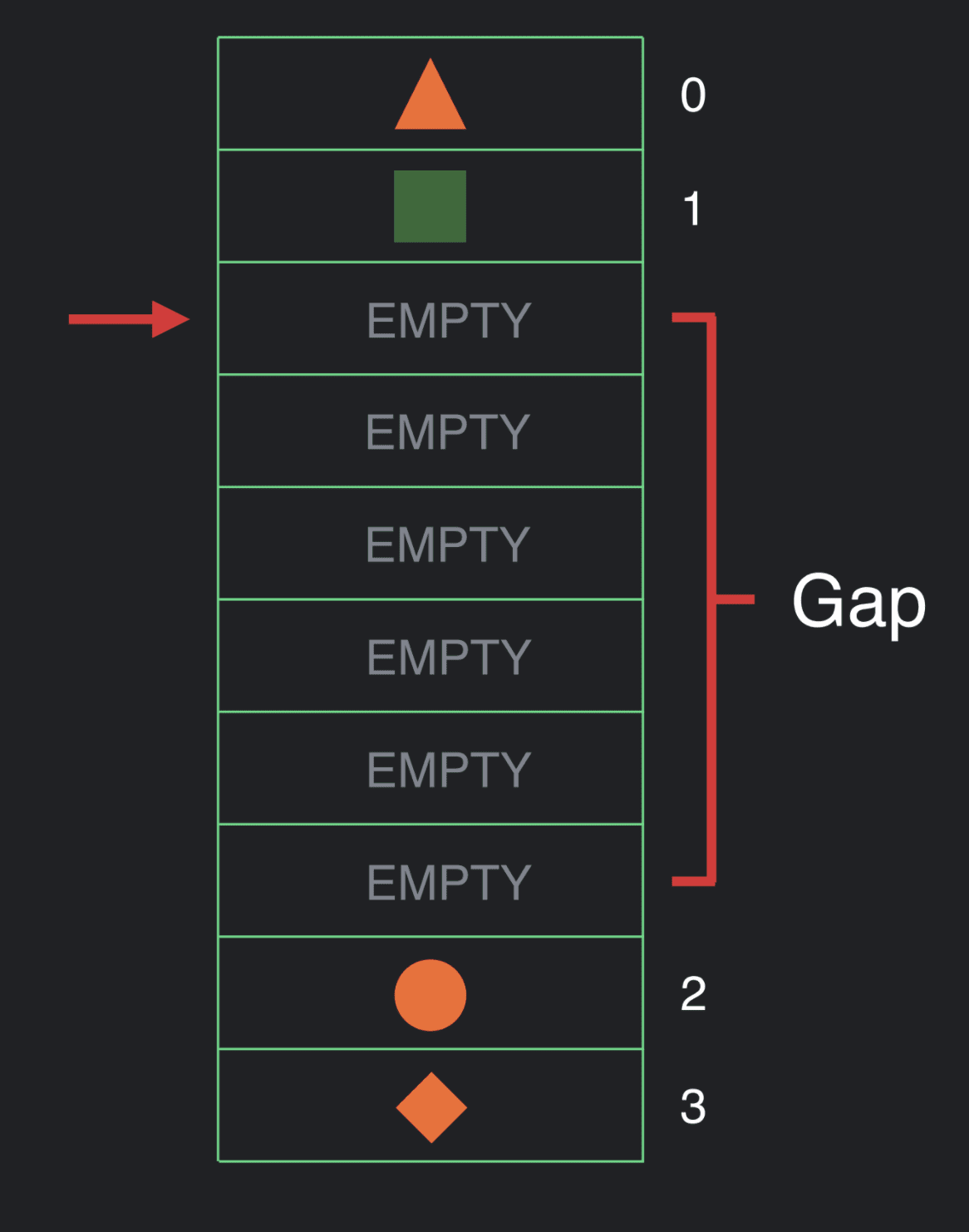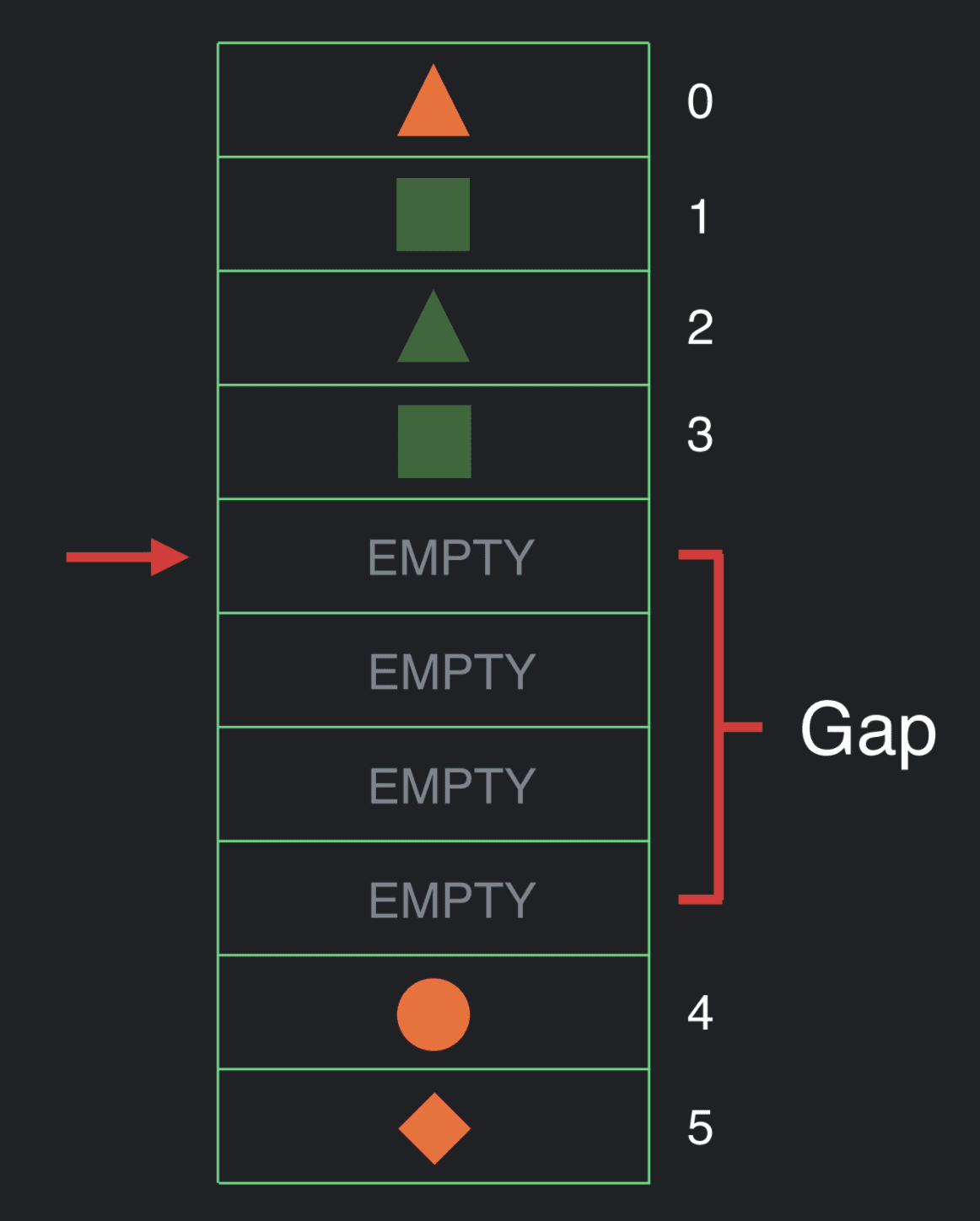``````@Composable
fun Counter() {
var count by remember { mutableStateOf(0) }
Button(
text="Count: \$count",
onPress={ count += 1 }
)
}
``````

``````fun Counter(\$composer: Composer) {
\$composer.start(123)
var count by remember { mutableStateOf(0) }
Button(
text="Count: \$count",
onPress={ count += 1 }
)
\$composer.end()
}
``````

``````fun Counter(\$composer: Composer) {
\$composer.start(123)
var count by remember(\$composer) { mutableStateOf(0) }
Button(
\$composer,
text="Count: \$count",
onPress={ count += 1 },
)
\$composer.end()
}
``````

• Composer.start 被调用并存储了一个组对象 (group object)
• remember 插入了一个组对象
• mutableStateOf 的值被返回，而 state 实例会被存储起来
• Button 基于它的每个参数存储了一个分组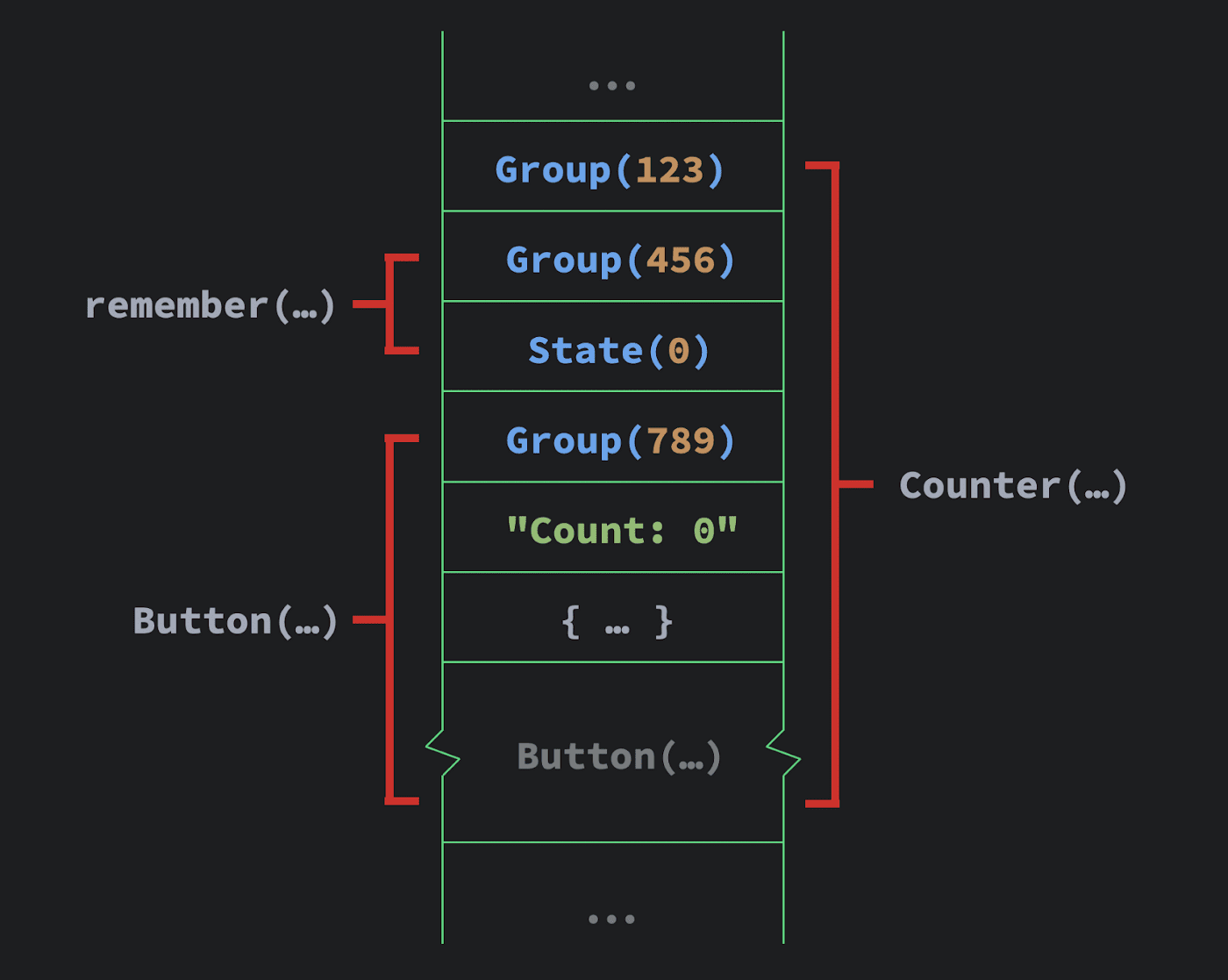``````@Composable fun App() {
val result = getData()
if (result == null) {
} else {
Body(result)
}
}
``````

``````fun App(\$composer: Composer) {
val result = getData()
if (result == null) {
\$composer.start(123)
\$composer.end()
} else {
\$composer.start(456)
Body(result)
\$composer.end()
}
}
``````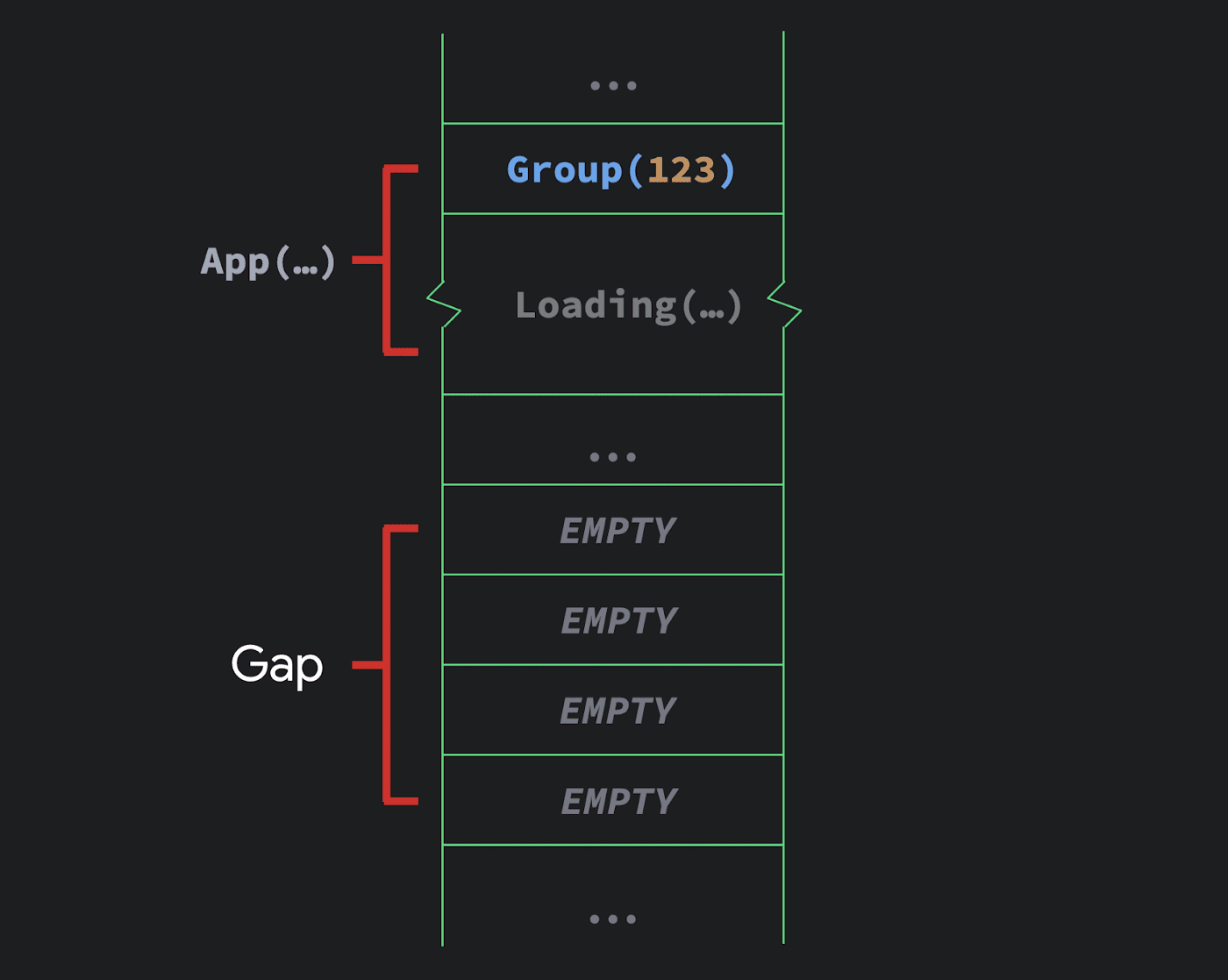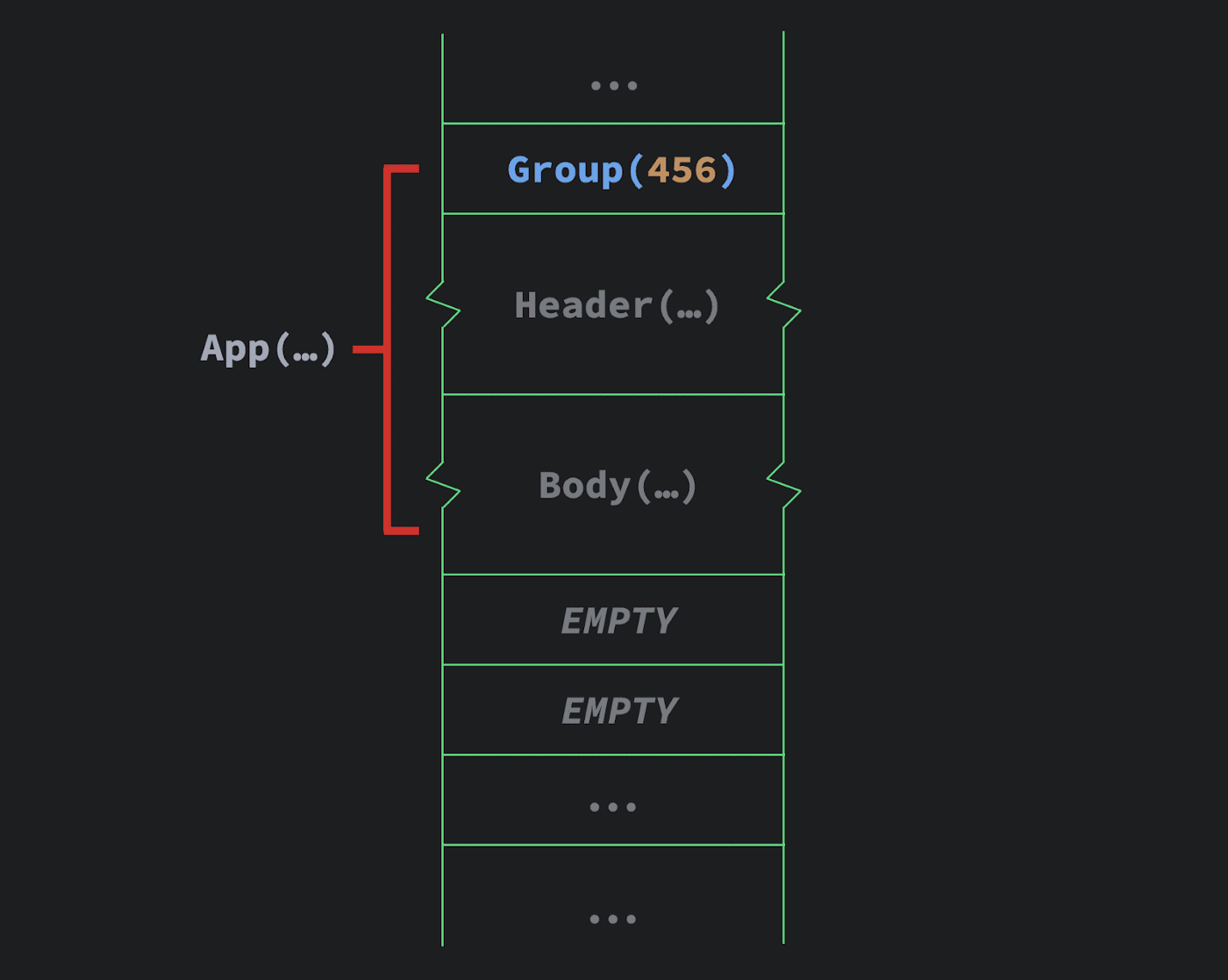## Positional Memoization (位置记忆化)

``````@Composable
fun App(items: List<String>, query: String) {
val results = items.filter { it.matches(query) }
// ...
}
``````

``````@Composable
fun <T> remember(vararg inputs: Any?, calculation: () -> T): T
``````

``````@Composable fun App() {
val x = remember { Math.random() }
// ...
}
``````

## 存储参数

``````@Composable fun Google(number: Int) {
number=number,
street="Amphitheatre Pkwy",
city="Mountain View",
state="CA"
zip="94043"
)
}

number: Int,
street: String,
city: String,
state: String,
zip: String
) {
Text("\$number \$street")
Text(city)
Text(", ")
Text(state)
Text(" ")
Text(zip)
}
``````

Compose 将 Composable 函数的参数存储在插槽表中。在本例中，我们可以看到一些冗余：Address 调用中添加的 “Mountain View” 与 “CA” 会在下面的文本调用被再次存储，所以这些字符串会被存储两次。

``````fun Google(
\$composer: Composer,
\$static: Int,
number: Int
) {
\$composer,
0b11110 or (\$static and 0b1),
number=number,
street="Amphitheatre Pkwy",
city="Mountain View",
state="CA"
zip="94043"
)
}
``````

``````fun Address(
\$composer: Composer,
\$static: Int,
number: Int, street: String,
city: String, state: String, zip: String
) {
Text(\$composer, (\$static and 0b11) and ((\$static and 0b10) shr 1), "\$number \$street")
Text(\$composer, (\$static and 0b100) shr 2, city)
Text(\$composer, 0b1, ", ")
Text(\$composer, (\$static and 0b1000) shr 3, state)
Text(\$composer, 0b1, " ")
Text(\$composer, (\$static and 0b10000) shr 4, zip)
}
``````

``````fun Google(
\$composer: Composer,
number: Int
) {
if (number == \$composer.next()) {
\$composer,
number=number,
street="Amphitheatre Pkwy",
city="Mountain View",
state="CA"
zip="94043"
)
} else {
\$composer.skip()
}
}
``````

Composer 知道快进至需要恢复的位置的距离。

## 重组

``````fun Counter(\$composer: Composer) {
\$composer.start(123)
var count = remember(\$composer) { mutableStateOf(0) }
Button(
\$composer,
text="Count: \${count.value}",
onPress={ count.value += 1 },
)
\$composer.end()
}
``````

``````\$composer.end()?.updateScope { nextComposer ->
Counter(nextComposer)
}
``````

## 最后

### 版权声明